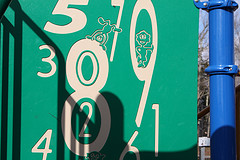# What Is A Compatible Number?Compatible numbers are numbers which are well matched for the purpose of doing mathematical sums. The purpose of using compatible numbers is to get a rough estimate of an answer to a question so that you can be sure that you have worked correctly when you try to calculate an exact answer.

Compatible numbers are useful in estimating the sum, difference, product or quotient of a problem. A compatible number will tend to be one that is very close to the number in the problem, but one which is more convenient and easy to use.

An example of when you might want to use compatible numbers might be when you are required to estimate the answer to the question 29 ÷ 6.5

In this example, the compatible numbers you could use instead of 29 and 6.5 could be 28 and 7. This is because 28 ÷ 7 = 4.

As a result of doing this estimation using compatible numbers, you now know roughly what the answer to the problem 29 ÷ 6.5 is likely to be. If we work out the exact answer to the question, we find out that the actual quotient is 4.46. We can be fairly confident that this is the correct answer as a result of the estimation using compatible numbers that we have already done.

thanked the writer.

A compatible is a number that is easy to use when answering a arithmetic question. A example
is like 53+67. The compatible number for 53 would be 50 and the compatible number for 67
would be 70.

thanked the writer.
A compatible number is one that is near the actual number, but is easier to use for addition, subtraction, multiplication, or division. In other words, it is easy to use for estimating the answer to an arithmetic problem.

Example
38 + 23
A compatible number for 38 might be 40
A compatible number for 23 might be 20 (or 25)

The sum of the compatible numbers would be 60 (or 65). So you know the answer will be near there.

If you choose 40 and 20, you know that one of your compatible numbers is a little too high, and the other is a little too low. So your estimate of the answer (40+20=60) will be pretty close. (Your estimators are high by 2 and low by 3, so you know your answer is low by 1. It is really 61, not 60.)

If you choose 40 and 25, you know that both of your compatible numbers are a little too high, so your answer will be a little more high. As it turns out, both "estimates" are high by 2, so the estimated answer (65) is high by 2+2=4. The actual result is 61 = 65-4.

thanked the writer.
When you round to the nearest ten, hundred, and thousand. For example, 23 would be 20 and 42 would be 40.
thanked the writer.
Defenition of a compatible number ~ Compatible numbers are numbers that look nice or friendly with each other when we do mental calculation to estimate a product, but especially a division

Some examples of compatible numbers when doing division are 400 and 10, 36 and 6, 2400 and 12, and 64 and 8

2400 and 12 are compatible because when doing this division (2400/12), we can quickly divide 24 by 12 to get 2 and put two zeros at the end to get 200

Some examples of compatible numbers when doing multiplication are 200 and 40, 1100 and 40, 25 and 4.

1100 and 40 are compatible because we can quickly do this multiplication by multipling 11 and 4 to get 44 and add three zeros at the end to get 44000
thanked the writer.
Numbers that are close to the original number and that make addition, subtraction, multiplication or division easier.  For example, compatible numbers to 23 and 51 are 25 and 50.
thanked the writer.

I may be mistaken, but actually a compatible number is easier than that.

Say you are adding 3 + 5 + 6 + 7 + 4

If you combine 3 + 7 = 10 and  6 + 4 = 10

you then have 10 + 10 + 5 = 25

thanked the writer.

Compatible numbers are numbers that get along well. For example:
2400 divided by 7  will be 342 with a remainder (not really sure). Well, how about we make 2400 to 2100 or even 2800 divided by 7? 2100 divided by 7 equals 300 with no remainder!  Plus you probably can do this mentally since 3*7=21,you can't really divide 7 by 2400 in your mind as quick this is why this is called compatible numbers!

If you still don't get it heres a website that explains what compatible numbers are and gives you some practice if needed.

thanked the writer.